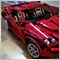# Calculating max drawdown in xx candles help - page 2425

Peter Kaiza:

I've amended  (LPrice>LLPrice) , should  the (HPrice>HHPrice) sign  be amended?

No. When checking whether a value is greater than max, always use >. Conversely, when checking whether a value is smaller than min, always use <. And HHPrice is the max, LLPrice is the min.844

Seng,

I'm sorry, The function is now returning 0.0 for downtrend throughout, what is wrong?

``` if (Direction<0)
{
for (int i=NumBars; i>0; i--)
{
double HPrice = iHigh(NULL,0,i);
double LPrice = iLow(NULL,0,i);

if (LPrice<LLPrice && LLPrice!=0 && InRetracement)
{
InRetracement = false;
LLPrice = 0;
double CurrRetracement = iHigh(NULL,0,HighBars[ArrayIdx])-iLow(NULL,0,LowBars[ArrayIdx]);
if (CurrRetracement>MaxRetracement || MaxRetracement==0)
MaxRetracement = CurrRetracement;
ArrayIdx++;
}

if (LPrice<LLPrice || LLPrice==0)
{
LLPrice = LPrice;
LowBars[ArrayIdx] = i;
}
else
if (HPrice>HHPrice || HHPrice==0)
{
HHPrice = HPrice;
HighBars[ArrayIdx] = i;
InRetracement = true;
}
}

if (InRetracement)
{
double CurrRetracement = iHigh(NULL,0,HighBars[ArrayIdx])-iLow(NULL,0,LowBars[ArrayIdx]);
if (CurrRetracement>MaxRetracement || MaxRetracement==0)
MaxRetracement = CurrRetracement;
}
}

return (MaxRetracement);
}```425

Peter Kaiza:

Seng,

I'm sorry, The function is now returning 0.0 for downtrend throughout, what is wrong?

Here's the error:

```         if (LPrice<LLPrice && LLPrice!=0 && InRetracement)
{
InRetracement = false;
LLPrice = 0;
double CurrRetracement = iHigh(NULL,0,HighBars[ArrayIdx])-iLow(NULL,0,LowBars[ArrayIdx]);
if (CurrRetracement>MaxRetracement || MaxRetracement==0)
MaxRetracement = CurrRetracement;
ArrayIdx++;
}
```

Should change to HHPrice = 0;844

Seng Joo Thio:

Here's the error:

Should change to HHPrice = 0;

There is still a problem with this function, returns o. Could it be this: if (Direction<0)?425

Peter Kaiza:

There is still a problem with this function, returns o. Could it be this: if (Direction<0)?

I don't know... I've tested already, should work. Anyway, I've attached my full code with both uptrend and downtrend - see if it differs from yours.

Files:844

Seng Joo Thio:

I don't know... I've tested already, should work. Anyway, I've attached my full code with both uptrend and downtrend - see if it differs from yours.

Seng,

Thank you very much it works , l did scratch my head quite a lot on this problem...... One more final question:

If for example I want to to recall  the retracement for the past  15 candles, should i leave the -1 on the oninit( or onstart on your code)   like below and on my code just use 1? Do I need to use -1 on both?

```  double Result = 0;

Result = FindBiggestRetracement(15,-1);

and on my code....

double RETRACE = FindBiggestRetracement(15, 1);```425

Peter Kaiza:

Seng,

Thank you very much it works , l did scratch my head quite a lot on this problem...... One more final question:

If for example I want to to recall  the retracement for the past  15 candles, should i leave the -1 on the oninit( or onstart on your code)   like below and on my code just use 1? Do I need to use -1 on both?

The 1 or -1 here represents the trend direction.
Or if you don't want to have to tell the function which direction the trend is heading, you can modify the function to determine based on a moving average, for example.844

Seng Joo Thio:
The 1 or -1 here represents the trend direction.
Or if you don't want to have to tell the function which direction the trend is heading, you can modify the function to determine based on a moving average, for example.

Ok to determine the trend drawdown in 15 bars,  in uptrend  or  downtrend  i've used this code  further down within ontick:

```

double TRENDUP = FindBiggestRetracement(15, 1);// Trend up drawdown

double TRENDDOWN = FindBiggestRetracement(15, -1);//Trend down drawdown```

Is this ok?425

Peter Kaiza:

Ok to determine the trend drawdown in 15 bars,  in uptrend  or  downtrend  i've used this code  further down within ontick:

Is this ok?

Yes.

To add comments, please log in or register